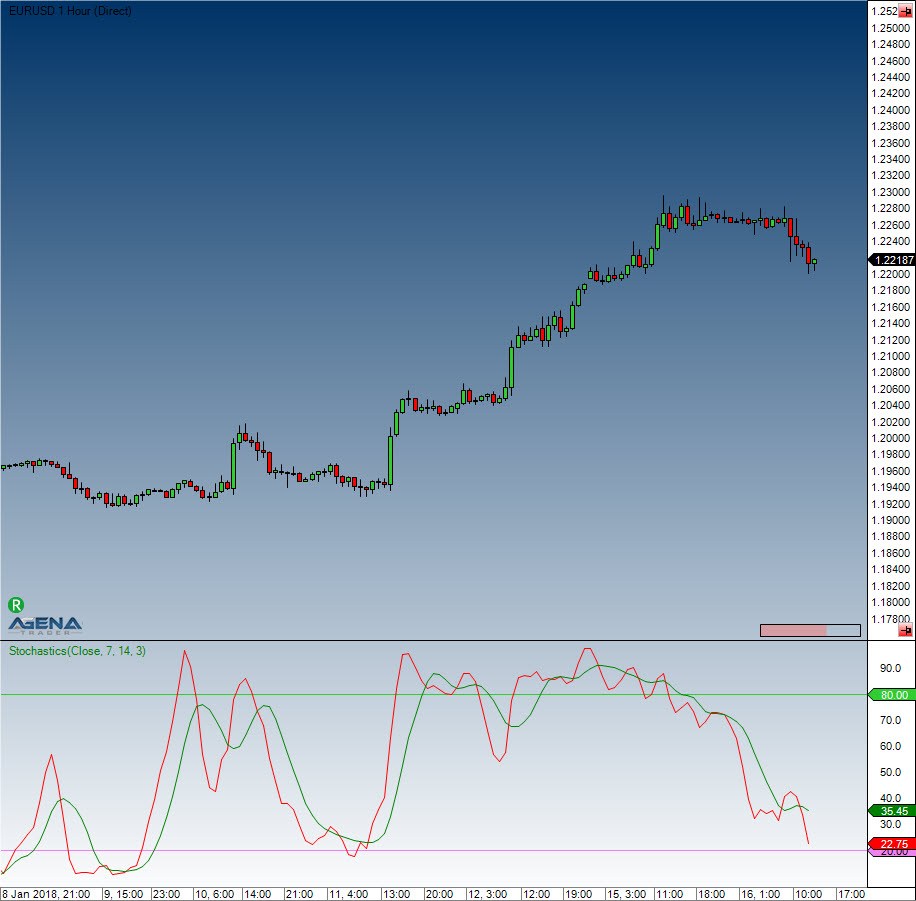Comment on page

# Stochastics

#### Description

The stochastic indicator was developed by George C. Lane in the 1950s. It belongs to the class “momentum indicators” (oscillators) and represents the current closing price in relation to a high or low area over a defined number of periods. The stochastic indicator is based on the observation that in an uptrend, the closing price is close to the daily high, while in a downtrend, the closing price is closer to the daily low. The stochastic indicator consists of two exponential Average Lines (%K and %D), both of which range between 0 and 100. There are two types of stochastic: the classic slow stochastic and the fast stochastic.
Fast stochastic: The %D line is the smoothing average of the %K line
Slow stochastic: The %D is the basis on which the smoothing average is calculated. The slow stochastic smoothes the fast stochastic, thus stabilizing the oscillator.

#### Interpretation

The closing prices found in the upper area imply an accumulation (buying pressure); the lower area represents selling pressure. The stochastic indicator is a classic oscillator, and is primarily used in sideways markets in order to determine the reversal points. Days with a stable trend will not provide the indicator with any relevant or valuable results/information.

#### Usage

Stochastics(int periodD, int periodK, int smooth)
Stochastics(IDataSeries inSeries, int periodD, int periodK, int smooth)
//For the %D line
Stochastics(int periodD, int periodK, int smooth).D[int barsAgo]
Stochastics(IDataSeries inSeries, int periodD, int periodK, int smooth).D[int barsAgo]
//For the %K line
Stochastics(int periodD, int periodK, int smooth).K[int barsAgo]
Stochastics(IDataSeries inSeries, int periodD, int periodK, int smooth).K[int barsAgo]

#### Return value

double
When using this method with an index (e.g. Stochastics(7,14,3)[int barsAgo] ), the value of the indicator will be issued for the referenced bar.

#### Parameters

inSeries Input data series for the indicator
periodD Number of periods to be used for the %K smoothing
periodK Number of bars included in the %K calculation
smooth Number of periods for the smoothing of %D

#### VisualizationStochastics

#### Example

//Output for the %K line
Print("The stochastic line %K is currently at: " + Stochastics(3, 14, 7).K);
//Output for the %D line
Print("The stochastic line %D is currently at: " + Stochastics(3, 14, 7).D);